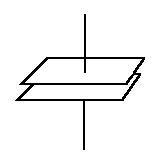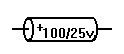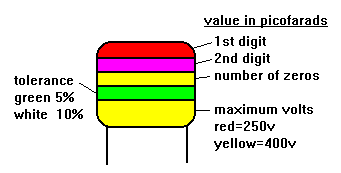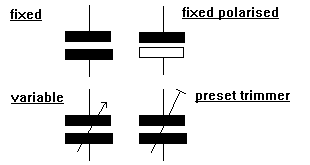Home > Electronics Tutorials > Components Tutorial > Capacitors Tutorial

# Capacitors Tutorial

Capacitors are basically two parallel metal plates separated by an insulator.This insulator is called the dielectric.

Capacitor types are named after the dielectric. Thus we have ceramic, mica, polyester, paper air capacitors etc.

Capacitors can be charged up and store electricity, similar to a car battery. This can be a hazard if they are charged up to high voltages. If it is necessary, capacitors with large charges should be discharged via a resistor to limit the discharge current. DC current cannot flow through a capacitor since the dielectric forms an open circuit.

Capacitors come in all shapes and sizes and are usually marked with their value. Values are measure in Farads. Values in Farads are unusual. Most capacitor values are measured in microfarads, nanofarads or picofarads. See the page on Value multipliers to find out more about this.They are often marked with their maximum working voltage. The voltage across the terminals must not exceed this value. It is OK to use a voltage below the maximum value.

Some capacitors such as electrolytic and tantalums are polarised. This means that they must be fitted the correct way round. They are marked to indicate polarity.

Some values are indicated with a colour code similar to resistors. There can be some confusion.
A 2200pf capacitor would have three red bands. These merge into one wide red band.Some values are marked in picofarads using three digit numbers. The first two digits are the base number and the third digit is a multiplier.

For example, 102 is 1000 pF and 104 is 100,000 pF = 100 nF = 0.1 uF.

To find the total value of capacitors in parallel (that is connected across each other) their values are added. To find the total value if they are in series (that is in line with each other) then the following formula is used.

1/C total =1/C1 + 1/C2 + 1/C3 etc

Variable capacitors are available in which the value can be adjusted by controlling the amount of overlap of the plates or the distance between them.There is a type of diode called the Varicap diode with similar characteristics.